Next: Incompressible Aerodynamics Up: Incompressible Boundary Layers Previous: Approximate Solutions of Boundary

# Exercises

1. Fluid flows between two non-parallel plane walls, towards the intersection of the planes, in such a manner that ifis measured along a wall from the intersection of the planes then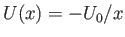, where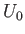is a positive constant. Verify that a solution of the boundary layer equation (8.35) can be found such thatis a function of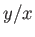only. Demonstrate that this solution yields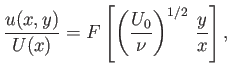where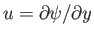, and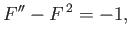subject to the boundary conditions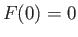and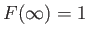. Verify that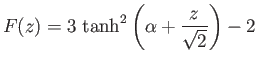is a suitable solution of the previous differential equation, where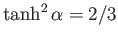.

2. A jet of water issues from a straight narrow slit in a wall, and mixes with the surrounding water, which is at rest. On the assumption that the motion is non-turbulent and two-dimensional, and that the approximations of boundary layer theory apply, the stream function satisfies the boundary layer equation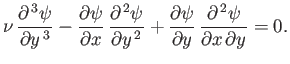Here, the symmetry axis of the jet is assumed to run along the-direction, whereas the-direction is perpendicular to this axis. The velocity of the jet parallel to the symmetry axis is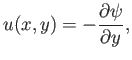where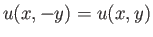, and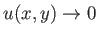as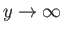. We expect the momentum flux of the jet parallel to its symmetry axis,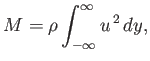to be independent of.

Consider a self-similar stream function of the formDemonstrate that the boundary layer equation requires that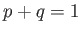, and that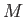is only independent ofwhen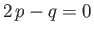. Hence, deduce that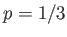and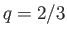.

Suppose that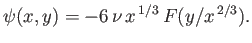Demonstrate that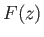satisfies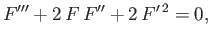subject to the constraints that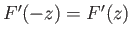, and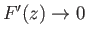as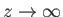. Show that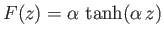is a suitable solution, and that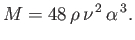3. The growth of a boundary layer can be inhibited by sucking some of the fluid through a porous wall. Consider conventional boundary layer theory. As a consequence of suction, the boundary condition on the normal velocity at the wall is modified to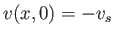, where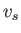is the (constant) suction velocity. Demonstrate that, in the presence of suction, the von Kármán velocity integral becomes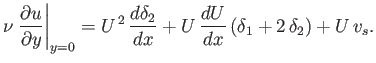Suppose that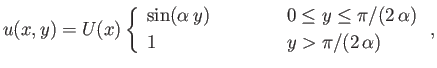where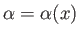. Demonstrate that the displacement and momentum widths of the boundary layer are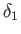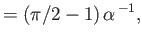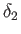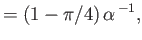respectively. Hence, deduce that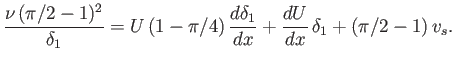Consider a boundary layer on a flat plate, for which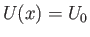. Show that, in the absence of suction,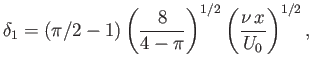but that in the presence of suction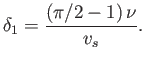Hence, deduce that, for a plate of length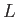, suction is capable of significantly reducing the thickness of the boundary layer when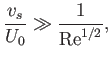where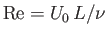.Next: Incompressible Aerodynamics Up: Incompressible Boundary Layers Previous: Approximate Solutions of Boundary
Richard Fitzpatrick 2016-03-31